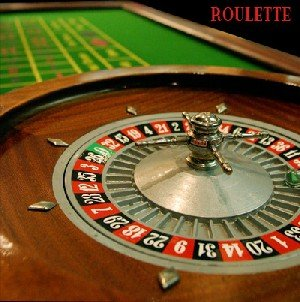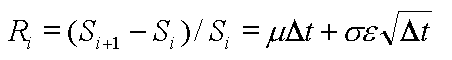# Calculating VaR using Monte Carlo Simulation

Monte Carlo Simulations correspond to an algorithm that generates random numbers that are used to compute a formula that does not have a closed (analytical) form – this means that we need to proceed to some trial and error in picking up random numbers/events and assess what the formula yields to approximate the solution. Drawing random numbers over a large number of times (a few hundred to a few million depending on the problem at stake) will give a good indication of what the output of the formula should be.It is believed actually that the name of this method stems from the fact that the uncle of one of the researchers (the Polish mathematician Stanislaw Ulam) who popularized this algorithm used to gamble in the Monte Carlo casino and/or that the randomness involved in this recurring methodology can be compared to the game of roulette.

We will now look at how Monte Carlo simulation can be applied to Value at Risk.

As we know, Monte Carlo Simulations correspond to an algorithm that generates random numbers that are used to compute a formula that does not have a closed (analytical) form – this means that we need to proceed to some trial and error in picking up random numbers/events and assess what the formula yields to approximate the solution. Drawing random numbers over a large number of times (a few hundred to a few million depending on the problem at stake) will give a good indication of what the output of the formula should be.

In the section, we will present the algorithm, and apply it to compute the VaR for a sample stock.

Computing VaR with Monte Carlo Simulations is very similar to Historical Simulations. The main difference lies in the first step of the algorithm – instead of using the historical data for the price (or returns) of the asset and assuming that this return (or price) can re-occur in the next time interval, we generate a random number that will be used to estimate the return (or price) of the asset at the end of the analysis horizon.

### Step 1 – Determine the time horizon t for our analysis and divide it equally into small time periods, i.e. dt = t/n).

For illustration, we will compute a monthly VaR consisting of twenty-two trading days. Therefore n = 22 days and $\delta t$ = 1 day. In order to calculate daily VaR, one may divide each day per the number of minutes or seconds comprised in one day – the more, the merrier.

The main guideline here is to ensure that $\delta t$ is large enough to approximate the continuous pricing we find in the financial markets. This process is called discretization, whereby we approximate a continuous phenomenon by a large number of discrete intervals.

### Step 2 – Draw a random number from a random number generator and update the price of the asset at the end of the first time increment.

It is possible to generate random returns or prices. In most cases, the generator of random numbers will follow a specific theoretical distribution. This may be a weakness of the Monte Carlo Simulations compared to Historical Simulations, which uses the empirical distribution. When simulating random numbers, we generally use the normal distribution.

In this article, we use the standard stock price model to simulate the path of a stock price from the ith day as defined by:Where:

• Ri is the return of the stock on the ith day
• Si is the stock price on the ith day
• Si+1 is the stock price on the i+1th day
• $\mu$ is the sample mean of the stock price
• $\delta t$ is the timestep
• $\sigma$ is the sample volatility (standard deviation) of the stock price
• $\epsilon$ is a random number generated from a normal distribution

At the end of this step/day ($\delta t$ = 1 day), we have drawn a random number and determined Si+1 since all other parameters can be determined or estimated.

### Step 3 – Repeat Step 2 until reaching the end of the analysis horizon T by walking along the N time intervals.

At the next step/day ($\delta t$ = 2), we draw another random number and determine Si+2 from Si+1 using the same equation. We repeat this procedure until we reach T and can determine Si+T. In our example, Si+22 represents the estimated (terminal) stock price in one month time of the sample share.

### Step 4 – Repeat Steps 2 and 3 a large number M of times to generate M different paths for the stock over T.

So far, we have generated one path for this stock (from i to i+22). Running Monte Carlo Simulations means that we build a large number M of paths to take account of a broader universe of possible ways the stock price can take over a period of one month from its current value (Si) to an estimated terminal price i+T. Indeed, there is no unique way for the stock to go from Si to Si+T. Moreover, Si+T is only one possible terminal price for the stock amongst an infinity. Indeed, for a stock price being defined on (a set of positive numbers), there is an infinity of possible paths from Si to Si+T.

It is an industry standard to run at least 10,000 simulations even if 1,000 simulations provide an efficient estimator of the terminal price of most assets. In this paper, we ran 1,000 simulations for illustration purposes.

### Step 5 – Rank the M terminal stock prices from the smallest to the largest, read the simulated value in this series that corresponds to the desired (1-$\alpha$)% confidence level (95% or 99% generally) and deduce the relevant VaR, which is the difference between Si and the ath lowest terminal stock price.

Let us assume that we want the VaR with a 99% confidence interval. In order to obtain it, we will need first to rank the M terminal stock prices from the lowest to the highest.

Then we read the 1% lowest percentile in this series. This estimated terminal price, Si+T1% means that there is a 1% chance that the current stock price Si could fall to Si+T1% or less over the period in consideration and under normal market conditions.

If Si+T1% is smaller than Si (which is the case most of the time), then Si - Si+T1% will corresponds to a loss. This loss represents the VaR with a 99% confidence interval.

In the next post, we will show you an example where we will apply the above steps to calculate the value at risk of an asset.

#### Lesson Resources

This bundle contains spreadsheets to help calculate value-at-risk in excel.